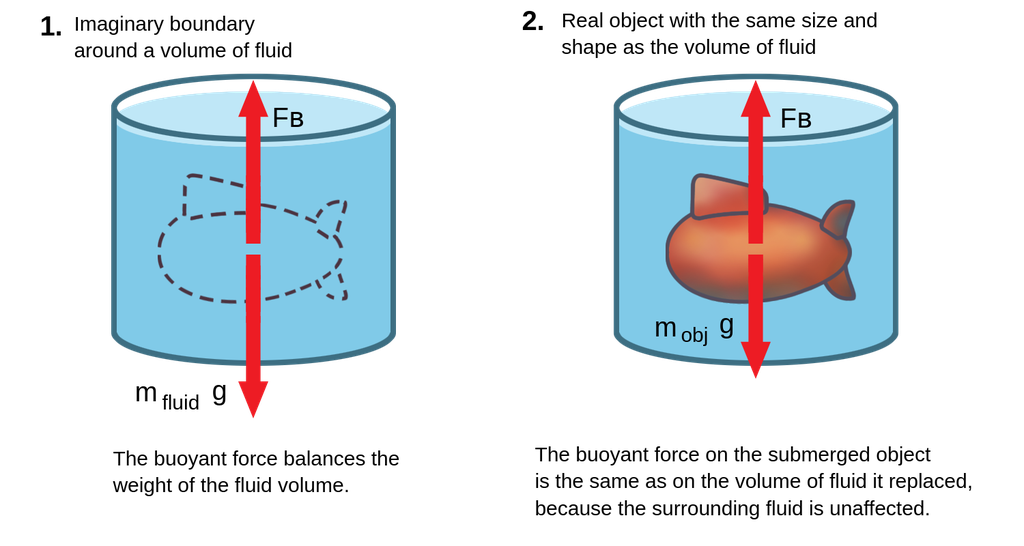Around 246 B.C., the Greek scientist Archimedes discovered that an object totally or partially immersed in a fluid, experiences an upward buoyant force FBequal to the weight of the fluid it displaces. This makes sense because that same volume of fluid was in equilibrium with its surroundings before the object was placed in the fluid (see Figure 1).

If the submerged volume of the object is Vsubm, the mass of fluid it displaces is given by fluid density · submerged volume, i.e. mdisp = ρfluid · Vsubm, and therefore the weight of the fluid displaced is mdisp · g. So we can write:

Buoyant force FB = mdisp · g = ρfluid · Vsubm · g

It is important to note that the only object property that affects the buoyant force is its volume - not what it is made of or its shape. For example, a solid metal ball and a hollow plastic ball, of the same volume, both held underwater experience equal buoyant forces.

Of course, as long as there is gravity (g), the object in the fluid always has the weight force pulling down on it, and the buoyant force pushing up on it. How these two forces compare determines whether the object sinks or floats.Figure 1: Archimedes Principle